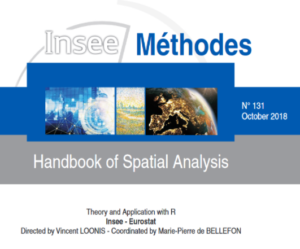Select Page

# INSEE Handbook of Spatial Analysis

by | Jan 24, 2022

Keywords
Principle 5spatial analysis, handbook, open source, R
Description
The purpose of this spatial analysis handbook is to answer the questions faced by research teams at statistical institutes: what use should be made of these new geolocated data sources? In what cases should their spatial dimension be taken into account? How should spatial statistical and econometric methods be applied? In contrast to existing manuals, its teaching principle has been expressly designed according to the issues specific to statistical institutes: the examples of application use data collected by public statistical institutes and the emphasis is placed on practice and the importance of parameter selection.

The theoretical foundations are explored in sufficient depth to enable an understanding of the subtleties in the practical implementation of methods, referring readers interested in understanding extensions of a higher technical level to specialised works. The handbook was prepared by the National Statistical Institute of France (INSEE).

The Handbook of Spatial Analysis is divided into four parts and fourteen chapters. The first three chapters match the stages one would follow to carry out a study with spatial data: describing the location of the observations, measuring spatial interactions and applying the appropriate model.

Each chapter explains the theoretical foundations, gives practical applications based on data coming from public statistical institutes, and displays how to use the R statistical software to carry out the computations.